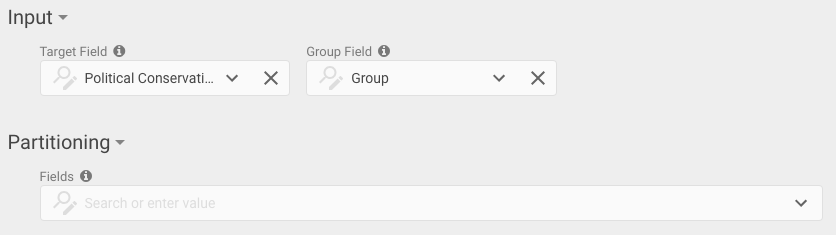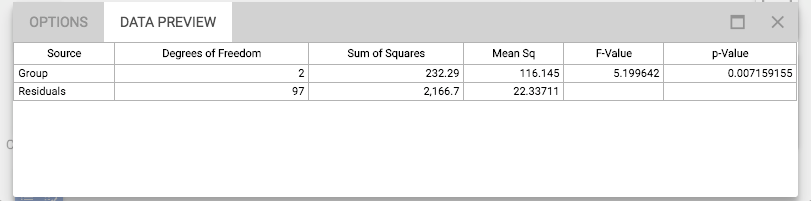# One Way ANOVA

Modified on Tue, 25 Feb 2020 at 04:05 PM

# In Brief

What: One Way ANOVA (Analysis of Variance) tests to see if groups of data have the same underlying source, meaning that their average values and variation are equivalent. The block provides some technical information associated with the statistical method, such as the sum of squares (a measure of variation) within each group and between each group.

The most important value the test produces is the p-value which in this case should be interpreted as the probability that all of the groups have the same underlying data source.

Typical Scenario: One way ANOVA tests are very commonly used in academia and life science, but also have some applications in business to test the difference between different fields of data.

## Case Study

### Workflow### OptionsTypical workflow and block options

Using Political Conservatism data different electoral colleges (in this case simply labeled A, B and C). Suppose we want to test to see if political conservatism is the same across the different subjects, One Way ANOVA is an effective method of doing this.

### Input DataSample of dataset

### Output

The following workflow is set up, and the block only requires the fields with the electoral college and field of interest to be selected. The workflow then produces the following output:Output data

The results display each of the components that are used to arrive at the p-value. Degrees of Freedom, Sum of Squares and Mean Sq all come from specific sources. Where the source is “Group” the values have been within each of the groups, where the source is “Residuals” the values have been found from between groups. The sum of square is effectively a measure of the variation from each of these two sources.

The F-value and p-value are the results of the overall test, the F-value a the “test statistic” which is used to find the p-value. The p-value can be interpreted as the probability that all of the groups have the same underlying data source.